# Physics - Vector Math and Operations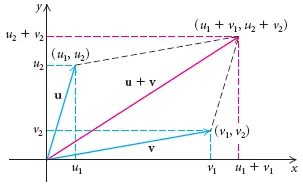Hello it's a me again drifter1! Today we continue with Physics getting into Vector Math and Operations between Vectors. Vectors are used in everything that has to do with Physics and are really important. That's why this post will be only about Vectors. We talked about Vector Spaces in Linear Algebra Mathematics, but there are some things that I don't talked about and are mostly used in Physics and that we will cover today! So, without further do, let's get started!

## Vectors

As I already said last time in my quick Introduction to this Series, we use physics quantities to describe the phenomena around us. But, most of the phenomena can't be described by a value only and need a direction of appliance also. This takes us back to the topic of Vectors...

Vectors are arrows that point from one point to another and describe the direction of movement and the length (meter) of the Vector describes by how much we move in each of the x, y or even z coordinates in 1-, 2- or 3-dimensional space.

This is how we symbolize a vector in a 2-dimensional space: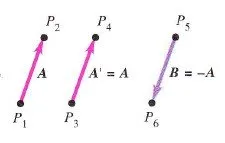A is a vector that goes from P1 to P2.

A' is a vector equal to the vector A, cause it has the same meter and direction, but it  not exactly the same as A, cause the points P3 and P4 are not equal to P1 and P2.

B is the negative of A (-A), cause it has the same meter, but the opposite direction.

The meter/length of A is symbolized as |A|, which is the same as saying the absolute value of A and is always positive!

This meter is equal to the square root of the coordinates squared:

|A| = root( x^2 + y^2 + z^2 + ...)  if we start of (0, 0, 0, ....)

If the starting point is not 0 then we do the following:

|A| = root ( (x2-x1)^2 + (y2-y1)^2 + (z2-z1)^2 + ...)

where (x1, y1, z1, ...) is the start point and (x2, y2, z2, ...) is the end point.

A vector and a physics quantity can also never be equal to each other! We can't say that 16kg = 5km the same way as we can't say that 2 apples are equal to 5 oranges.

An important operation between vectors is the addition.

Suppose that a point mass (object) follows the path of a vector A and then the path of B.

This looks like that: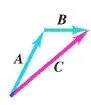What if we first follow B and then A?Well, the result is exactly the same!

So, if we add those vectors using the rule of the parallelogram and the result C = A + B we end up with exactly the same position as before...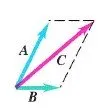So, when adding wo vectors we use this rule and that way we also know that the order of addition doesn't matter. This means that A + B = B + A and the Commutative Law applies to vector addition.

Generalizing it we can also apply vector addition to more then 2 vectors by applying it in groups of 2. The Associative Law applies and so the order doesn't matter!

So, if we want to add the vectors A, B and C we can do the following:

A + B + C = (A + B) + C = D + C = R

or

A + B + C = A + (B + C) = A + E = R

or even

A + B + C = (A + C) + B =  F + B = R

This looks like that: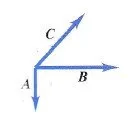A + B + C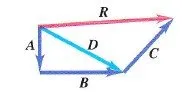(A + B) + C = D + C = R

### Vector Substraction

When substracting we simple add the negative vector and use the same exact technique as in addition. This means that if we want to find out what A - B equals we  get the negative vector of B and so -B and then add it to the vector A.

This looks like that:The Vectors A and B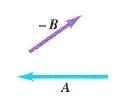Draw the negative of B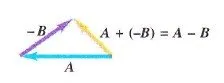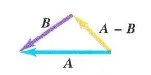The final result

Using this method we found out the direction and position of the vector in space and can now calculate the meter using the square root. But there is also another way. We can also use a simpler aproach by using the Pythagora Theorem if the angle is 90 degrees to find the meter of the result by using the meter of the building blocks of the addition. Using the tangent tanθ we can also find out the angle θ of the result vector to the xx' axis. We can also use the generalized Pythagora theorem for angles between the addition vectors that are not 90 degrees.

So, when adding 2 vectors A and B with meter 1 and 2 respectively and a 90 degree angle, then the result vector C will have a meter equal to root(1^2 + 2^2) = 2.24 and a tangent tanθ = 2/1 = 1 which means that we have an angle θ = 63,4 degrees to the xx' axis.

## Vector Components

Using the sinus and cosinus of the same angle θ we can do the opposite work to split a vector into 2, 3 or more other vectors. Those vectors that give us our source vector when added together are called vector components.

So, if A is a Vector in 2-dimensional space then we can write it as a sum of 2 vectors:

A = Ax + Aywhere Ax is the component in the x axis and Ay in the y axis.

Using the sinus and cosinus of angle θ we can find out the value of Ax and Ay.

Ax/A = cosθ and Ay/A = sinθ and so:

Ax = A cosθ and Ay = A sinθ

The meter of A is equal to the root(Ax^2 + Ay^2)

and we can calculate the angle using:

tanθ = Ay / Ax => θ = arctan(Ay/Ax)

## Unit Vectors

Another interesting topic are the unit vectors in each axis. The unit vector of a specific axis has a meter of 1. The x axis has a unit vector called i and the y axis a unit vector called j. We also use k as a unit vector of the z axis when we are in 3-dimensional space.

Using these unit vectors we can now write a vector A as components like that:

A = Ax i + Ay j

where Ax = Ax i and Ay = Ay j

We can also write the addition of two vectors using unit vectors like that:

R = A + B = (Ax i + Ay j) + (Bx i + By j) = (Ax + Bx)i + (Ay + By)j = Rx i + Ry j

The same also applies to 3-dimensional space, where we will use the unit vector k

As you can clearly see, the unit vectors help us to distinguish which vectors are in which axis and so we add only those together that are in a specific axis to get a result that contains one vector for each axis, where some axis may also not contain a value.

## Vector Multiplication

The last and final topic we will cover today is Vector Multiplication. There are 2 basic types of multiplication. The so called scalar or dot product and the cross product.

Scalar product:

The scalar or dot product is not a vector and represents a scalar quantity. To get the dot product the vectors need to have a common starting point and we also need the angle φ between those two vectors.

So, the scalar product of A*B is:

A*B = A B cosφ = |A| |B| cosφ

As you can see this value is not a vector and can be positive, negative or even zero, when the degree is equal to 90. When the degree is equal to 0 we have the maximum value and the scalar product equals to the multiplication of the meters. When the degree is 180 we have the maximum negative value.

For the unit vectors we have that:

i*i = j*j = k*k = 1*1*cos 0 = 1

i*j = i*k = j*k = 1*1*cos 90 = 0

When multiplying across multiple dimensions we keep only the product in the same axis and so:

A*B = (Ax i + Ay j + Az k) * (Bx i + By j + Bz k) = AxBx + AyBy + AzBz

Cross product:

The cross product of two vectors is a vector that "points out" of the two vectors.

This looks like that: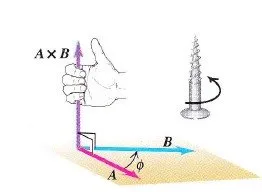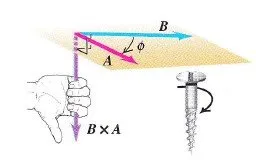This is used a lot in 3-dimensional physics that we will get into much later on!

The cross product is calculated using:

C = AxB = ABsinφ

where φ is the angle when going from A to B and we always use the smallest angle possible so that the angle is between 0 and 180 degrees. This means that if A and B have a degree of φ = 0 or 180 the cross product equals 0, which means that they are (anti)parallel to each other.

To find out the direction of the vector we use the Right hand rule that you can read about here.

The cross product AxB is not equal to the cross product BxA, cause AxB = - BxA!

The unit vector cross products look like that:

ixi =jxj = kxk = 0

ixj = -jxi = k

jxk = -kxj = i

kxi = -ixk = j

This means that we will include the products in a different axis and so:

A x B = (Ax i + Ay j + Az k) x (Bx i + By j + Bz k) = Cx i + Cy j + Cz k

where:
Cx = AyBz - AzBy

Cy = AzBx - AxBz

Cz = AxBy - AyBx

That can be calculated simply by writing the cross product as an determinant: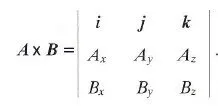Note that i x j = k is used in clockwise systems (the x, y, z we are all familiar with) and needs to be changed to i x j = - k if we get into counterclockwise systems.

And this is actually it and I hope that you learned something!

From next time on we will start getting into Classical Mechanics and Kinematics mostly!

Bye!

H2
H3
H4
3 columns
2 columns
1 column
1 Comment